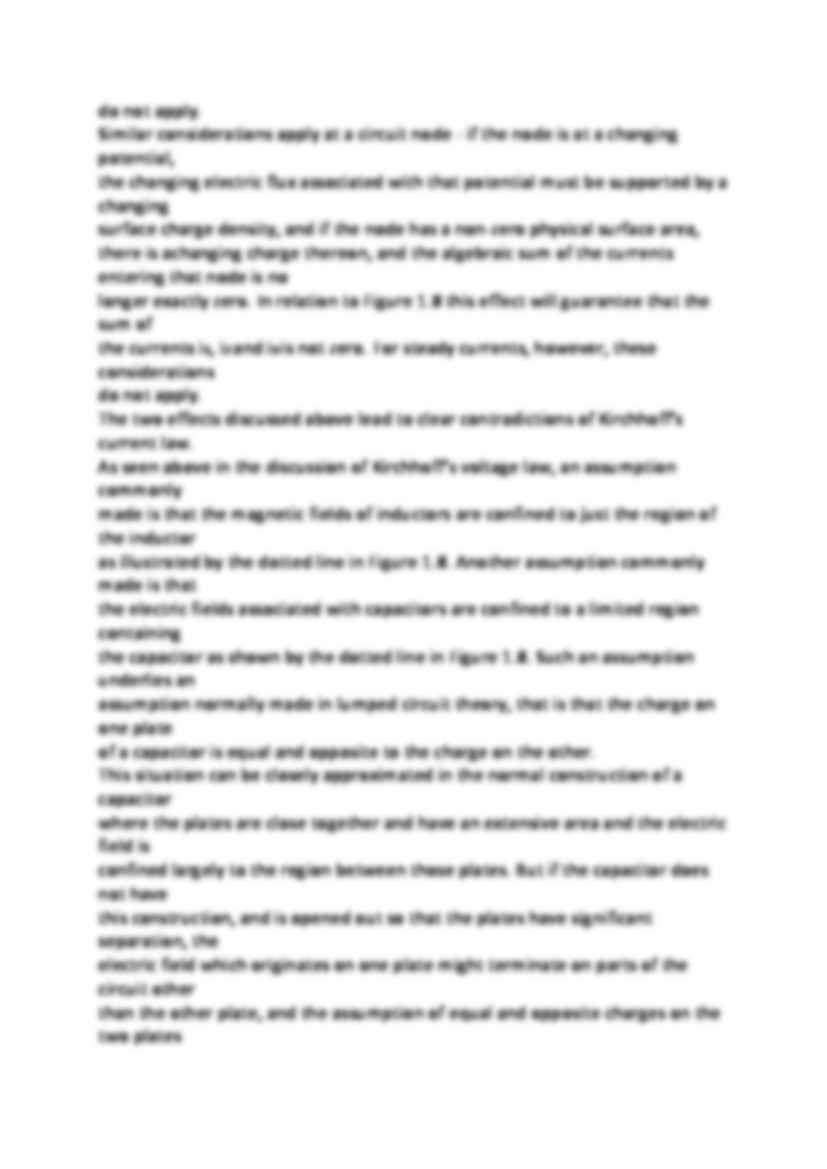# Kirchhoffs current law - wykład

Nasza ocena:

Wyświetleń: 518
Komentarze: 0

### Pobierz ten dokument za darmo

Podgląd dokumentu## Fragment notatki:

Kirchho ff s current law Kirchhoff's current law states that the algebraic sum of the currents entering a single
node of circuit is zero.
There are a number of assumptions underlying this statement. The first is that we
know where the currents are in the sense that the currents flow on wires, we know where
the wires are, and the current which flows into one end of a wire comes out the other. If
we were to use bare wires, and dip our circuit into a conducting fluid such as sea water,
none of this would be true - significant currents would leave the wires all along their length
and significant current density would flow with some unknown distribution throughout
the fluid.
Because living at the bottom of the ocean is for us uncomfortable, we do not commonly
do this, but an equivalent thing is happening when we consider the effects of displacement
current arising from changing electric fields between the wires. The electric flux density
which originates at one wire must be supported by an equal surface charge density on that
wire (whether it is insulated or not) and if the electric flux is changing in time, so will the
surface charge density. Because of the principle of conservation of charge, this changing
surface charge must come from somewhere, and it causes the current which flows along
the wire to leave behind little bits of charge along the length of that wire so that the
current entering one end of the wire is no longer equal to the current leaving at the other
end. In relation to Figure 1.8 this would ensure that current i4 entering the top-most wire
is not equal to the current i1 leaving it. For steady currents, however, these considerations
do not apply.
Similar considerations apply at a circuit node - if the node is at a changing potential,
the changing electric flux associated with that potential must be supported by a changing
surface charge density, and if the node has a non-zero physical surface area, there is a changing charge thereon, and the algebraic sum of the currents entering that node is no
longer exactly zero. In relation to Figure 1.8 this effect will guarantee that the sum of
the currents i1, i2 and i3 is not zero. For steady currents, however, these considerations
do not apply.
The two effects discussed above lead to clear contradictions of Kirchhoff's current law.
As seen above in the discussion of Kirchhoff's voltage law, an assumption commonly
made is that the magnetic fields of inductors are confined to just the region of the inductor
as illustrated by the dotted line in Figure 1.8. Another assumption commonly made is that
the electric fields associated with capacitors are confined to a limited region containing

(…)

… plates have significant separation, the
electric field which originates on one plate might terminate on parts of the circuit other
than the other plate, and the assumption of equal and opposite charges on the two plates
breaks down.
So we see that Kirchhoff's current law for a.c. circuits is an approximation, which
depends on a number of factors including the geometry normally adopted for the…
... zobacz całą notatkę

## Komentarze użytkowników (0)

Zaloguj się, aby dodać komentarz